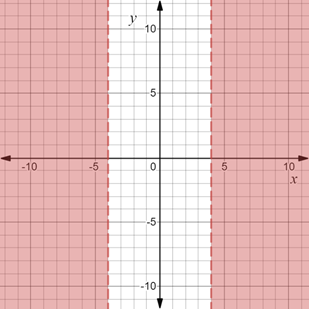# To graph : The region provided by the set { ( x , y ) | | x | &gt; 4 } .### Precalculus: Mathematics for Calcu...

6th Edition
Stewart + 5 others
Publisher: Cengage Learning
ISBN: 9780840068071### Precalculus: Mathematics for Calcu...

6th Edition
Stewart + 5 others
Publisher: Cengage Learning
ISBN: 9780840068071

#### Solutions

Chapter 1.8, Problem 29E
To determine

## To graph: The region provided by the set {(x,y)||x|>4} .

Expert Solution

### Explanation of Solution

Given information:

The set, {(x,y)||x|>4} .

Graph:

The graph of the region provided by the set {(x,y)||x|>4} is sketched in the coordinate plane.

Recall that |x|>a is equivalent to x>a or x<a .

Therefore, |x|>4 is equivalent to x>4 or x<4 .

Construct broken vertical lines x=4 and x=4 and shaded the region outside it.

The region obtained is provided below,Interpretation:

The region provided by the set {(x,y)||x|>4} is shaded region. The broken vertical line at x=4 and x=4 denote that they are not included in the region.The region consists of all those points whose x-coordinate does not lie between x=4 and x=4 . Therefore, shaded region is the region outside broken vertical lines x=4 and x=4 and these two vertical lines are not included in the region.

### Have a homework question?

Subscribe to bartleby learn! Ask subject matter experts 30 homework questions each month. Plus, you’ll have access to millions of step-by-step textbook answers!